# High School Math : Limits

## Example Questions

### Example Question #2 : Calculus I — Derivatives

Calculate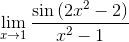.The limit does not exist.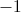Explanation:

Substitute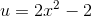to rewrite this limit in terms of u instead of x. Multiply the top and bottom of the fraction by 2 in order to make this substitution:

(Note that as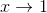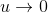.)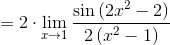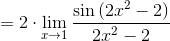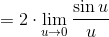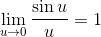, so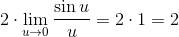, which is therefore the correct answer choice.

### Example Question #3 : Calculus I — Derivatives

Calculate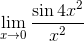The limit does not exist.Explanation:

You can substitute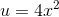to write this as: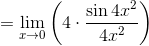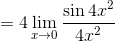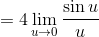Note that as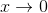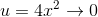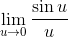, since the fraction becomes indeterminate, we need to take the derivative of both the top and bottom of the fraction.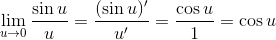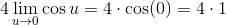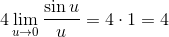, which is the correct choice.